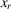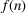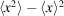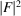International
Tables for
Crystallography
Volume B
Reciprocal space
Edited by U. Shmueli

International Tables for Crystallography (2006). Vol. B. ch. 2.1, p. 195   | 1 | 2 |

## Section 2.1.4.5. Non-independent variables

U. Shmuelia* and A. J. C. Wilsonb

aSchool of Chemistry, Tel Aviv University, Tel Aviv 69 978, Israel, and bSt John's College, Cambridge, England
Correspondence e-mail:  ushmueli@post.tau.ac.il

#### 2.1.4.5. Non-independent variables

| top | pdf |

The central-limit theorem, under certain conditions, remains valid even when the variables summed in equation (2.1.4.19)are not independent. The conditions have been investigated by Bernstein (1922, 1927); roughly they amount to requiring that the variables should not be too closely correlated. The theorem applies, in particular, when eachis related to a finite number,, of its neighbours, when the x's are said to bedependent. Thedependence seems plausible for crystallographic applications, since the positions of atoms close together in a structure are closely correlated by interatomic forces, whereas those far apart will show little correlation if there is any flexibility in the asymmetric unit when unconstrained. Harker's (1953) idea of `globs' seems equivalent todependence. Long-range stereochemical effects, as in pseudo-graphitic aromatic hydrocarbons, would presumably produce long-range correlations and makedependence less plausible. If Bernstein's conditions are satisfied, the central-limit theorem would apply, but the actual value ofwould have to be used for the variance, instead of the sum of the variances of the random variables in (2.1.4.19). Because of the correlations the two values are no longer equal.

French & Wilson (1978) seem to have been the first to appeal explicitly to the central-limit theorem extended to non-independent variables, but many previous workers [for typical references, see Wilson (1981)] tacitly made the replacement – in the X-ray case substituting the local mean intensity for the sum of the squares of the atomic scattering factors.

### ReferencesBernstein, S. (1922). Sur la théorème limite du calcul des probabilités. Math. Ann. 85, 237–241.Google ScholarBernstein, S. (1927). Sur l'extension du théorème limite du calcul des probabilités aux sommes de quantités dépendantes. Math. Ann. 97, 1–59.Google ScholarFrench, S. & Wilson, K. (1978). On the treatment of negative intensity observations. Acta Cryst. A34, 517–525.Google ScholarHarker, D. (1953). The meaning of the average offor large values of interplanar spacing. Acta Cryst. 6, 731–736.Google ScholarWilson, A. J. C. (1981). Can intensity statistics accommodate stereochemistry? Acta Cryst. A37, 808–810.Google Scholar